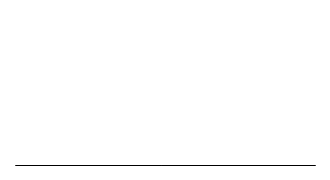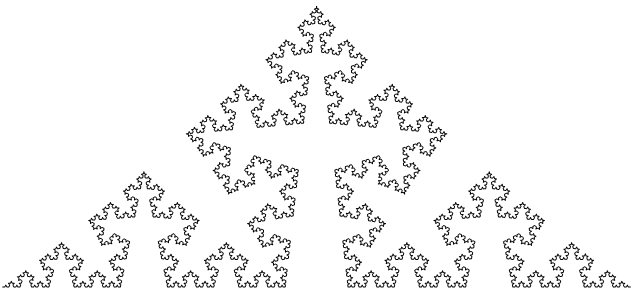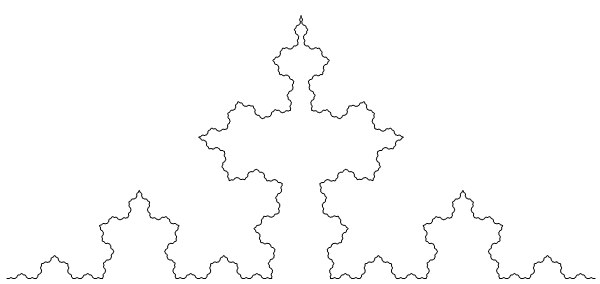# Quasifractals

One of the more familiar examples of fractal curves is the Koch snowflake. It is a closed curve of infinite length and Hausdorff dimension log(4)/log(3), homeomorphic to the unit circle. It bounds a region which, when combined in two different sizes, can tile the plane (as noted by Benoit Mandelbrot):The Koch snowflake can be constructed from three copies of the von Koch curve. This is a self-similar fractal curve, composed of four copies of itself, each scaled by$\sigma = \frac{1}{3}$. Varying the value of σ between ¼ and ½ yields a family of fractal curves, with Hausdorff dimension ranging from 1 (a straight line) to 2 (a spacefilling curve):In each of the curves, the same value of σ is used for all iterations. If, instead, we vary the value of σ, then the Hausdorff dimension is a function of the limiting value of σ (assuming the limit exists). In the following curve, σ successively takes on the values {1/4 + 1/5, 1/4 + 1/6, … , 1/4 + 1/n, …}, so the limiting value of σ is ¼, and the Hausdorff dimension is 1. Hence, this is not technically a fractal. However, an easy computation will verify that the length (product, over all iterations, of 4σ) is infinite.In other words, we have a non-fractal curve that nevertheless exhibits many of the same properties as fractal curves (infinite length, approximate self-similarity, etc.). On the other hand, if the values of σ converge to ¼ sufficiently quickly, then we obtain a curve of finite length.This entry was posted in Uncategorized. Bookmark the permalink.

### 2 Responses to Quasifractals

1.Kerry Mitchell says:

How is “sufficiently quickly” defined? If the quasi fractal case has sigma = 1/4 + 1/n, does the non-fractal case have sigma = 1/4 + 1/n^2 or some other power of n? If so, where is the threshold power of n between curves of finite and infinite length?

•apgoucher says:

Infinite length occurs if and only if the infinite product of (4 sigma) diverges, which occurs if and only if the infinite sum of (sigma – 1/4) diverges. Hence, your sigma = 1/4 + 1/n^2 has finite length, as does the curve obtained be replacing 2 with any other power greater than 1.